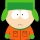Home
IT Knowledge
Inspiration
Languages
EN

# Python - print difference of two sets

0 points
Created by:Fletcher-Peralta
418

In this article, we would like to show you how to print the difference of two sets in Python.

## Practical example

In this example, we use `symmetric_difference_update()` to get the difference of `my_set1` and `my_set2`.

``````my_set1 = {'A', 'B', 'C'}
my_set2 = {'D', 'B', 'C'}

my_set1.symmetric_difference_update(my_set2)

print(my_set1)  # {'D', 'A'}``````

Output:

``{'D', 'A'}``